Homework Help Question & Answers

# Calculate buckling load for the elements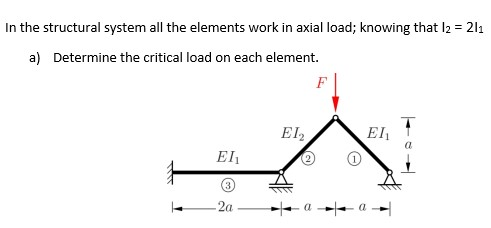In the structural system all the elements work in axial load; knowing that $$I_{2}=\left.2\I_{1}$$

a) Determine the critical load on each element.

Determine the critical buckling load
Alexander Ramirez

#### Homework Answers

Answer #1 ✔ Recommended Answer

If any query please let me know. Thanks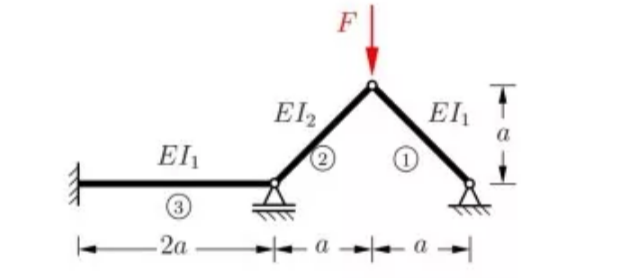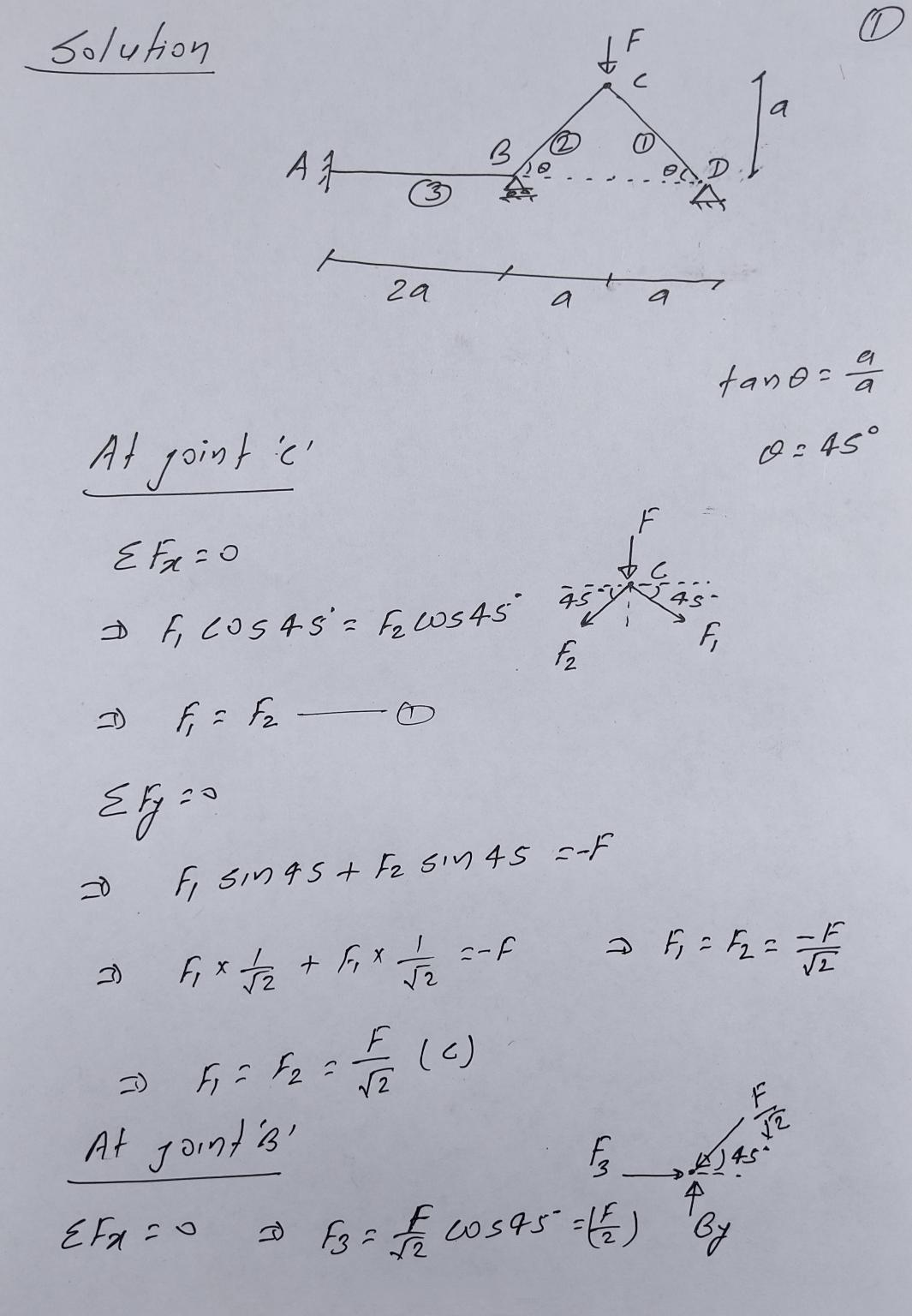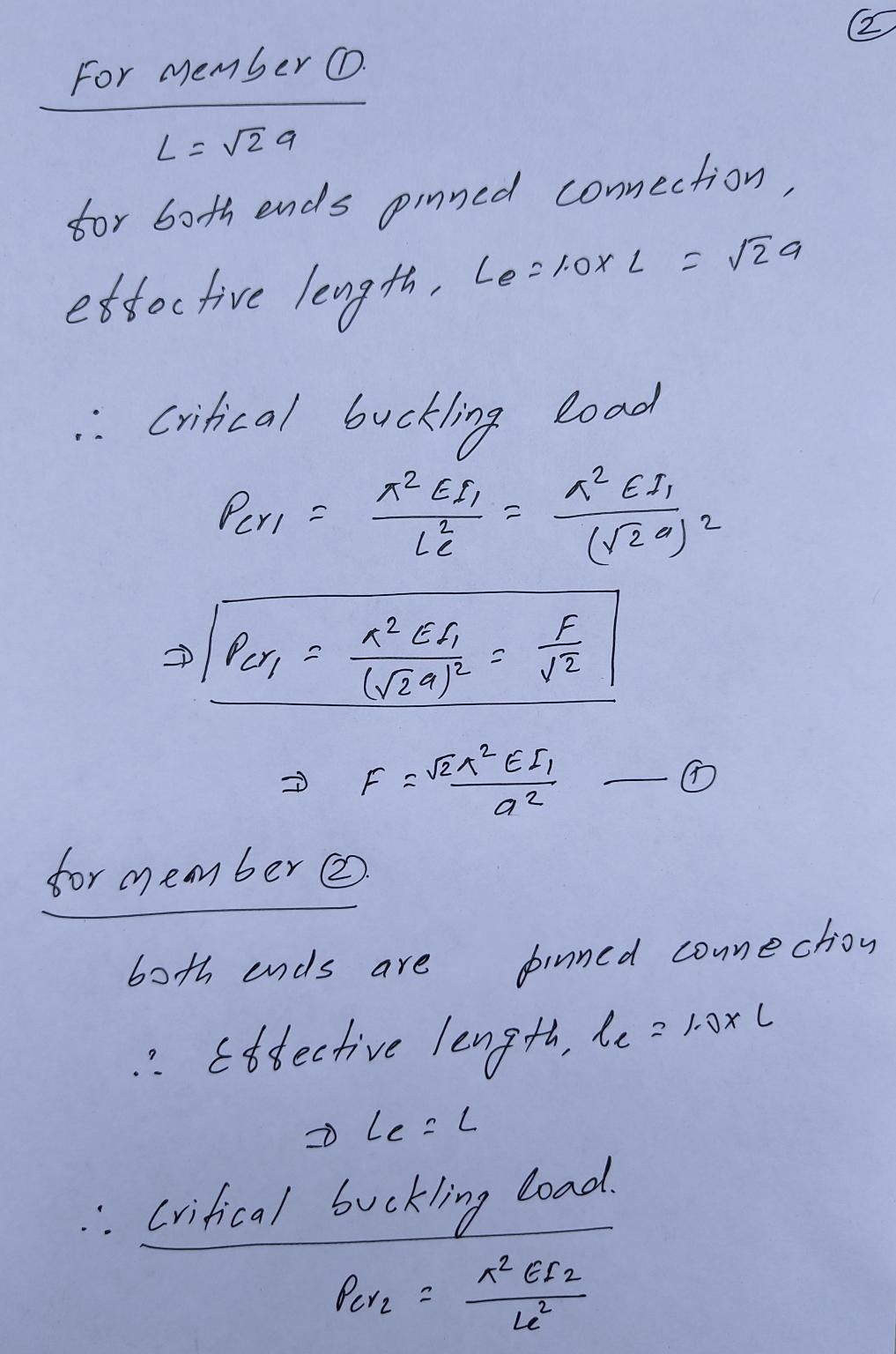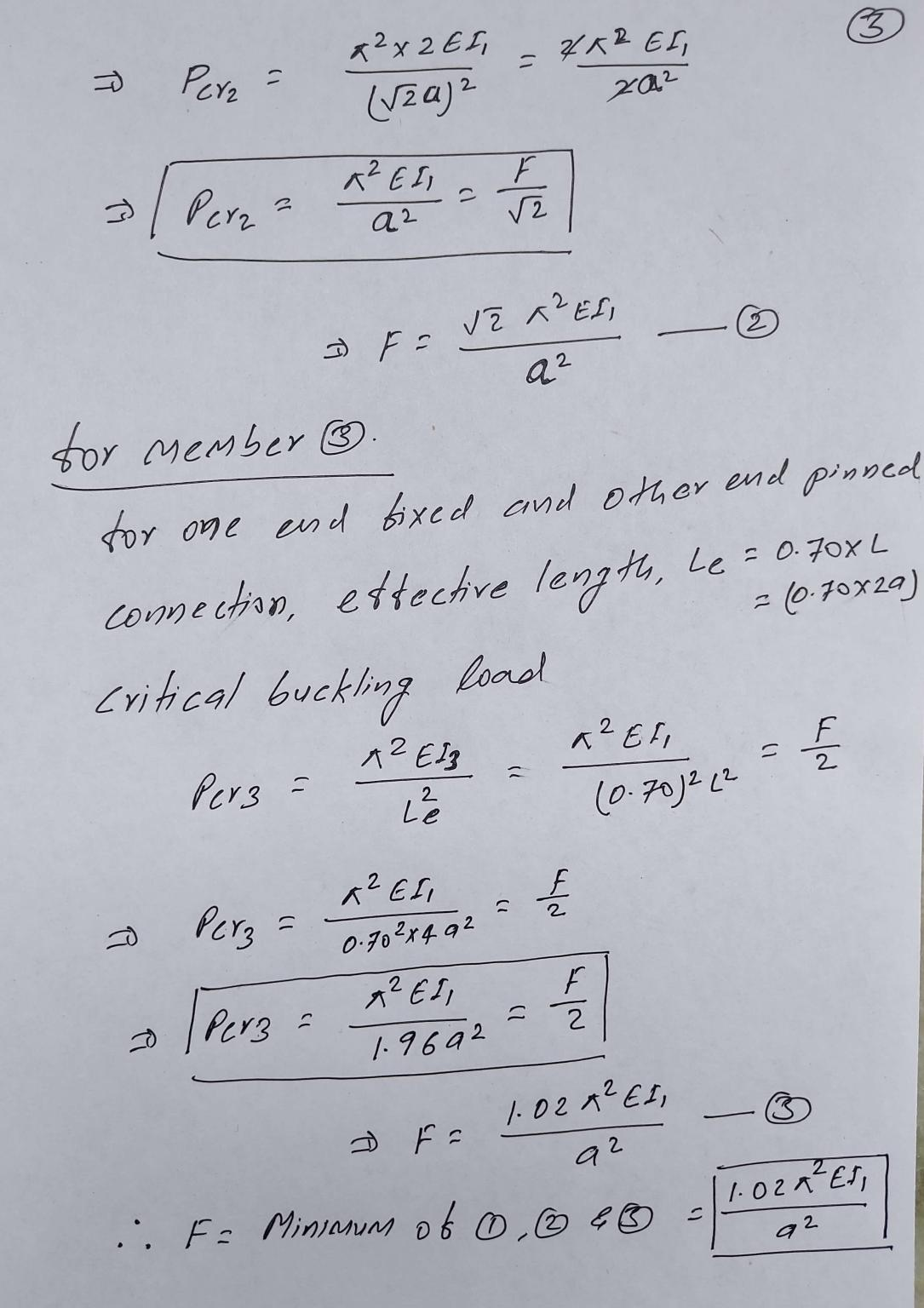in the critical load numerator, the letter before EI is the number pi????
Alexander Ramirez
wonderful answer ! Thank you so much. you've helped me too much
Alexander Ramirez
on the force of element 3, how did you calculate 1.02?
Alexander Ramirez
Add a comment
Know the answer?
Your Answer:

#### Post as a guest

Your Name:

What's your source?

#### Earn Coin

Coins can be redeemed for fabulous gifts.

Similar Homework Help Questions
• ### 1. The plane truss shown in Figure P3.10 is subjected to a downward vertical load at...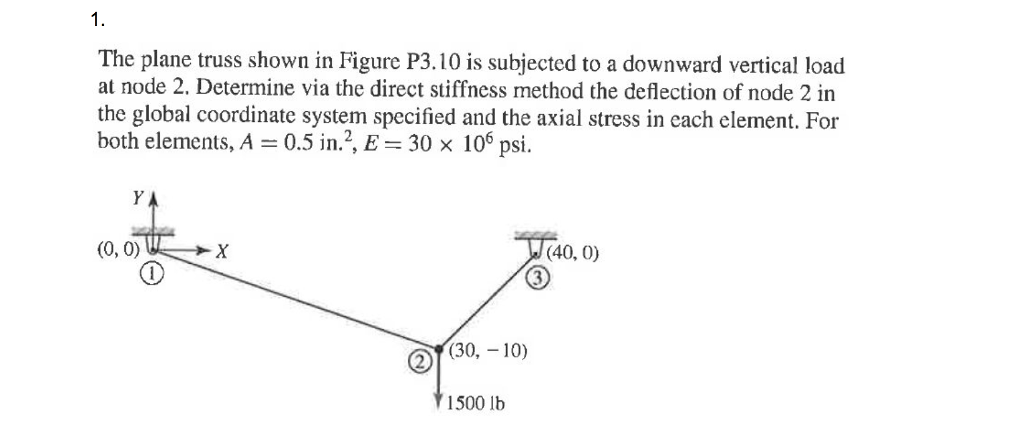1. The plane truss shown in Figure P3.10 is subjected to a downward vertical load at node 2. Determine via the direct stiffncss method the deflection of node 2 in the global coordinate system specified and the axial stress in each element. For both elements, A 0.5 in.2, E 30 x 106 psi. S1. (0,0) и х (40. 0) of30,-10) 1500 lb

• ### The frame below has wind load and dead as shown. Use w(Dead) = 6 kip/ft and...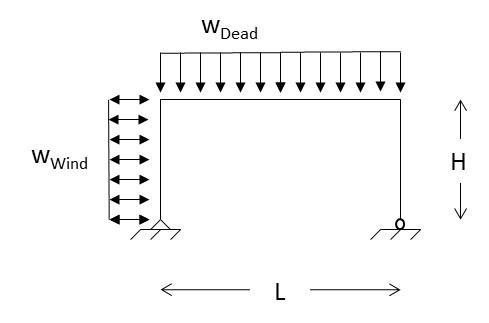The frame below has wind load and dead as shown. Use w(Dead) = 6 kip/ft and w(Live) = 3 kip/ft, L = 30 ft and H = 15 ft. The beams and columns have modulus of elasticity E of 29000 ksi and moment of inertias I(beam) = 2000 in4 and I(column) = 800 in4. Similarly they have cross-sectional areas A(beam) = 20 in2 and A(column) = 25 in2. Consider that the wind can act in both horizontal directions. Determine: The...

• ### Please and Thank You The buckling equation provides a critical load P = (ET Pe O...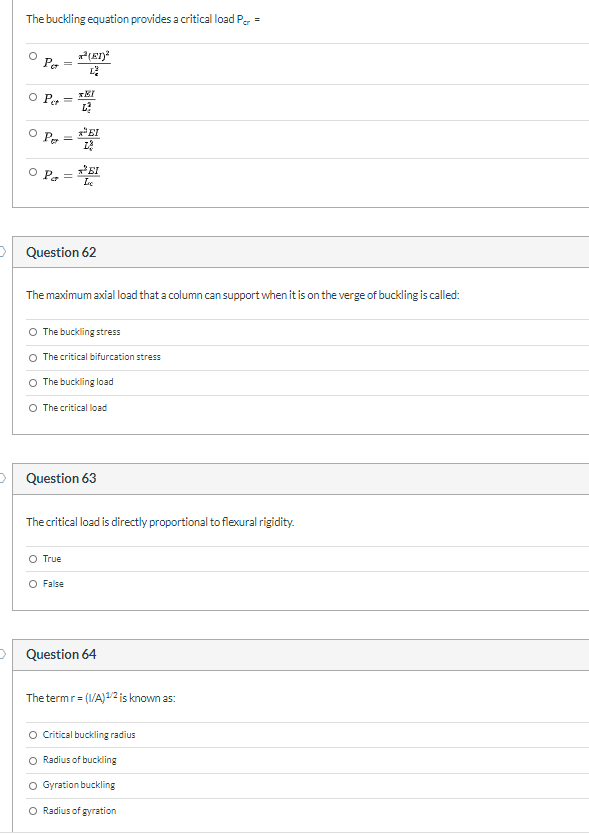Please and Thank You The buckling equation provides a critical load P = (ET Pe O Pet = he Per 51 22 OP= SI LE Question 62 The maximum axial load that a column can support when it is on the verge of buckling is called: The buckling stress The critical bifurcation stress The buckling load O The critical load Question 63 The critical load is directly proportional to flexural rigidity. O True O False Question 64 The termr =...

• ### Determine: a)the force in the left spring b)the force in the middle spring c)the axial force...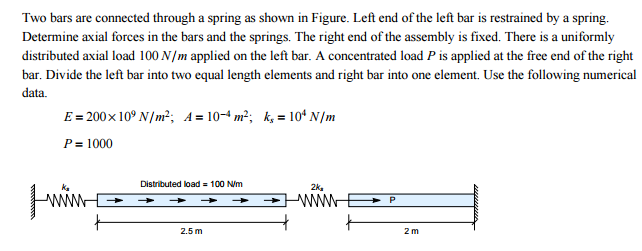Determine: a)the force in the left spring b)the force in the middle spring c)the axial force in the left half of the left bar d)the axial force in the right half of the left bar e)the axial force in the right bar Two bars are connected through a spring as shown in Figure. Left end of the left bar is restrained by a spring. Determine axial forces in the bars and the springs. The right end of the assembly is...

• ### Solve please. CMCE 2416 Elements of Structural Design - Concrete Assignment 4: Short Column with Axial...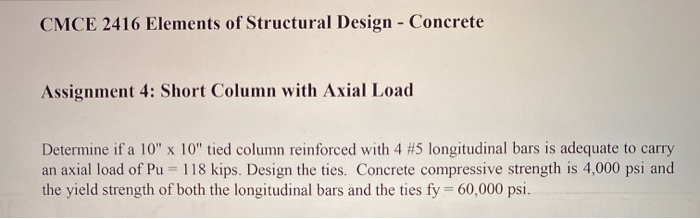Solve please. CMCE 2416 Elements of Structural Design - Concrete Assignment 4: Short Column with Axial Load Determine if a 10" x 10" tied column reinforced with 4 #5 longitudinal bars is adequate to carry an axial load of Pu = 118 kips. Design the ties. Concrete compressive strength is 4,000 psi and the yield strength of both the longitudinal bars and the ties fy = 60,000 psi.

• ### Critical Buckling Load--Spring Connection The leg in (a) acts as a column which can be modeled...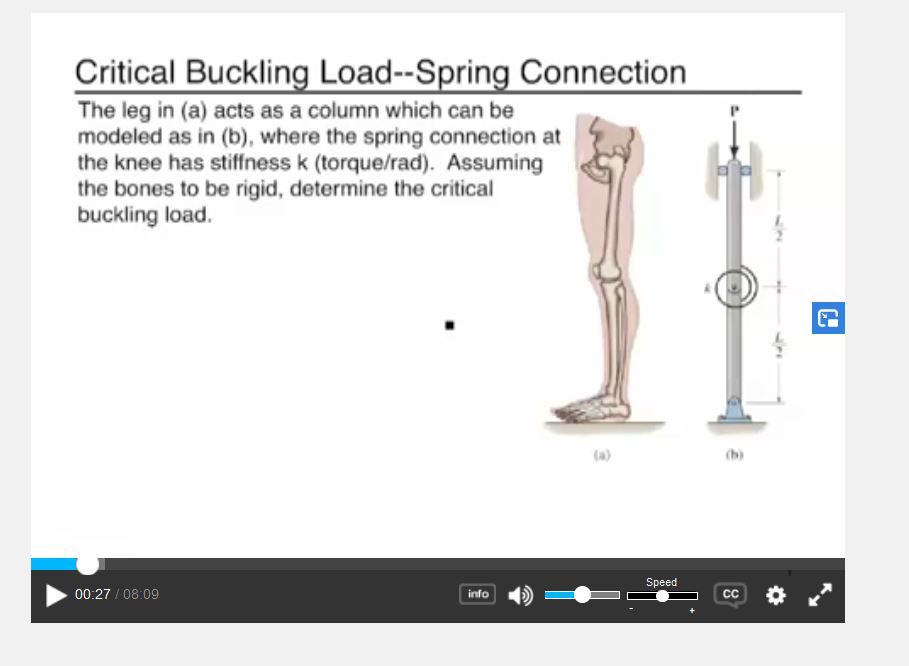Critical Buckling Load--Spring Connection The leg in (a) acts as a column which can be modeled as in (b), where the spring connection at the knee has stiffness k (torque/rad). Assuming the bones to be rigid, determine the critical buckling load. 2 Speed 00:27/08:09 info CC The critical load of a spring connection is: is a larger value than the pre-load state assumed to be a small angle approximation before buckling occurs found through the analysis of the FBD all...

• ### help Deduce the equations for all the nodes and calculate the load to which each element...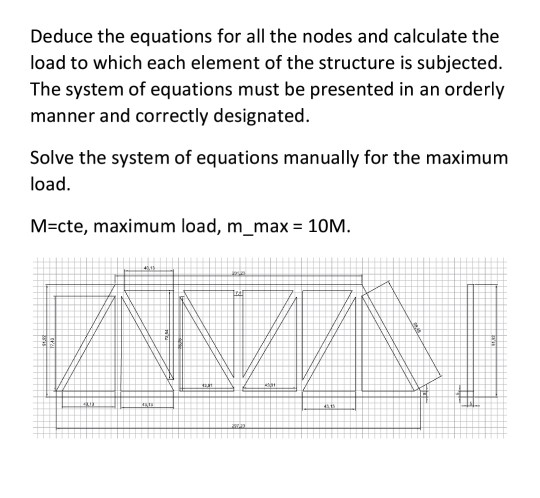help Deduce the equations for all the nodes and calculate the load to which each element of the structure is subjected. The system of equations must be presented in an orderly manner and correctly designated. Solve the system of equations manually for the maximum load. M=cte, maximum load, m_max = 10M. A.

• ### A tie rod (1) and a pipe strut (2) are used to support a 50-kN load,...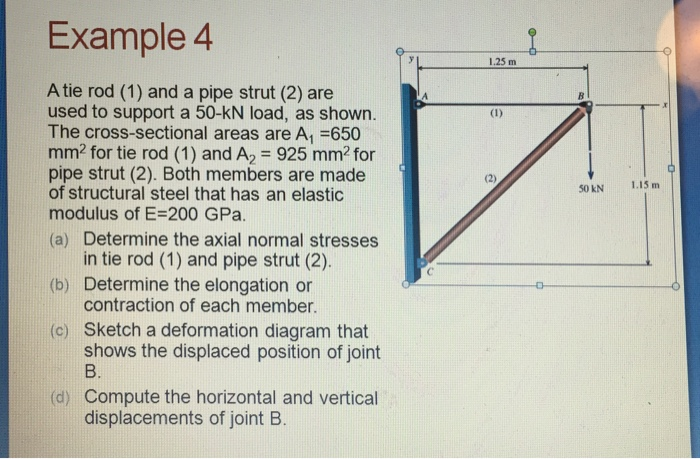A tie rod (1) and a pipe strut (2) are used to support a 50-kN load, as shown. The cross-sectional areas are A1 =650 mm2 for tie rod (1) and A2 = 925 mm2 for pipe strut (2). Both members are made of structural steel that has an elastic modulus of E=200 GPa. a) Determine the axial normal stresses in tie rod (1) and pipe strut (2). b) Determine the elongation or contraction of each member. c) Sketch a deformation...

• ### m Review Learning Goal: To use the formula for the critical load, i.e., the Euler buckling...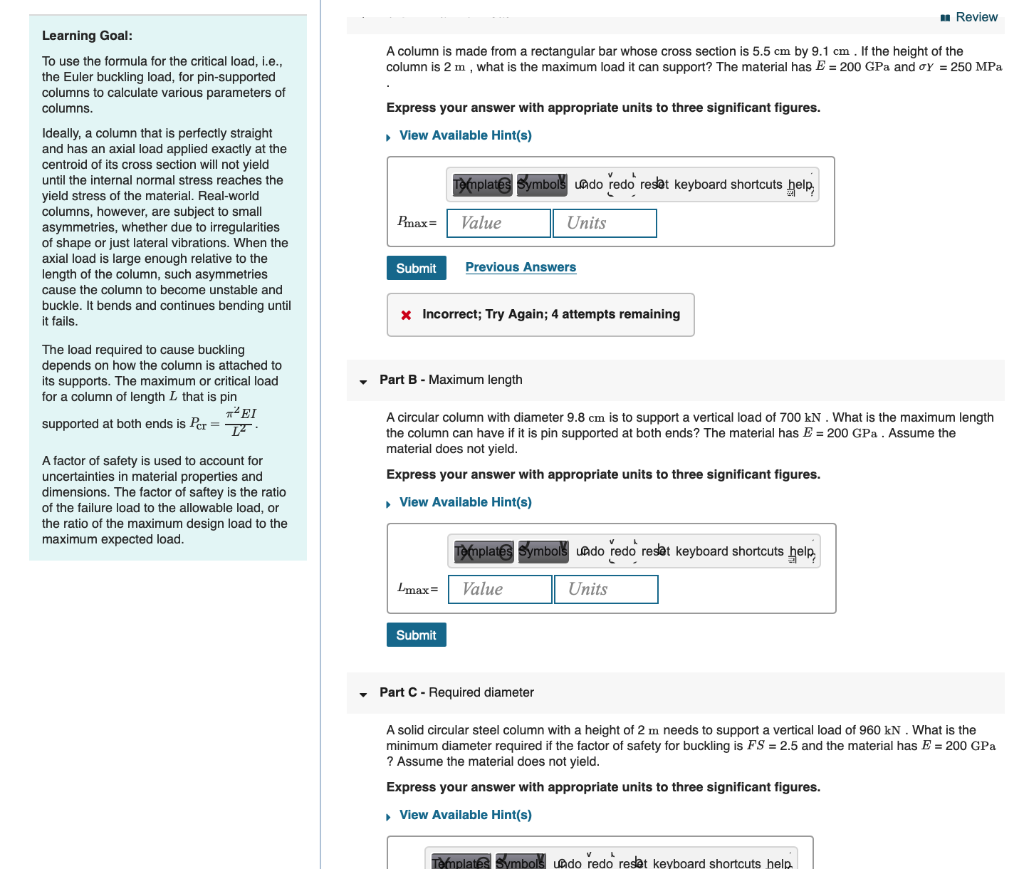m Review Learning Goal: To use the formula for the critical load, i.e., the Euler buckling load, for pin-supported columns to calculate various parameters of columns. A column is made from a rectangular bar whose cross section is 5.5 cm by 9.1 cm . If the height of the column is 2 m, what is the maximum load it can support? The material has E = 200 GPa and Oy = 250 MPa Express your answer with appropriate units to...

• ### Determine the critical buckling load for the rectangular aluminum alloy column AB. Set H = 5.9...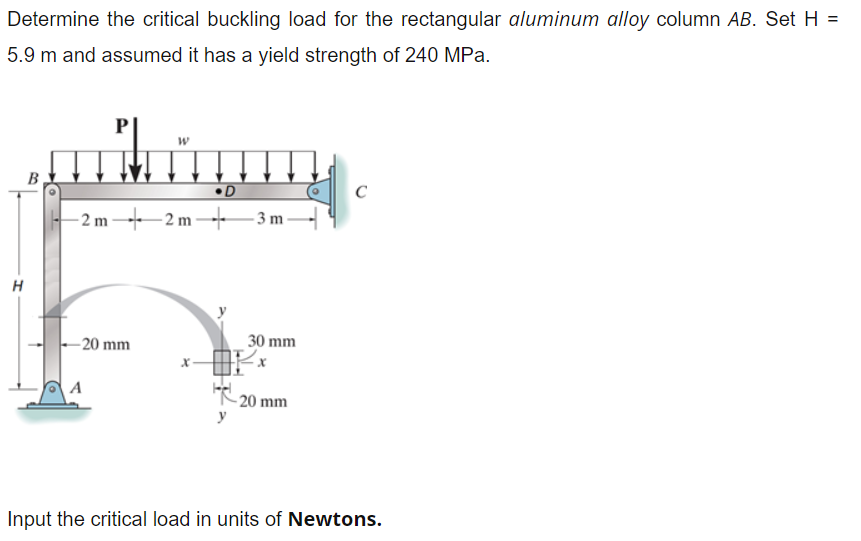Determine the critical buckling load for the rectangular aluminum alloy column AB. Set H = 5.9 m and assumed it has a yield strength of 240 MPa. D +2 m-42 m---3m-4 20 mm 30 mm 20 mm Input the critical load in units of Newtons.

Free Homework App

Scan Your Homework
to Get Instant Free Answers
Need Online Homework Help?

Get Answers For Free
Most questions answered within 3 hours.